# RRB ALP 2018 Practice Test Papers | Arithmetic Questions (Day-44)

Dear Aspirants, Here we have given the Important RRB ALP & Technicians Exam 2018 Practice Test Papers. Candidates those who are preparing for RRB ALP 2018 can practice these Arithmetic Questions to get more confidence to Crack RRB 2018 Examination.

[WpProQuiz 2233]

Click “Start Quiz” to attend these Questions and view Explanation

1. Govind is 48 years old. He is twice as old as his son Prem is now. How old was Prem seven years before?

(a) 16

(b) 17

(c) 13

(d) 18

1. What is the value of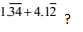(a) 133/ 90

(b) 371/ 90

(c) 5 219 990

(d) 5 461/990

1. A sum of money is divided among A, B, C and D in the ratio 3: 5: 8: 9 respectively. If the share of D is Rs. 1,872 more than the share of A, then what is the total amount of money of B & C together?

(a) Rs. 4,156

(b) Rs. 4,165

(c) Rs. 4,056

(d) Rs. 4,068

1. What approximate compound interest can be obtained on an amount of Rs.3,980 after 2 years at 8 p.c.p.a.?

(a) 650

(b) 680

(c) 600

(d) 662

1. By selling 8 dozen pencils, a shopkeeper gains the selling price of 1 dozen pencils. What is the gain?

(a) 12 1/ 2%

(b) 13 1 /7%

(c) 14 2/ 7%

(d) 87 1/ 2%

1. An aeroplane flies along the four sides of a square at the speeds of 200, 400, 600 and 800 km/h. Find the average speed of the plane around the field.

(a) 384 km/h

(b) 370 km/h

(c) 368 km/h

(d) None of these

1. A, B and C can do a work in 6, 8 and 12 days respectively. Doing that work together they get an amount of Rs. 1350. What is the share of B in that amount?

(a) Rs. 450

(b) Rs. 168.75

(c) Rs. 337.50

(d) Rs. 718.75

1. A and B started a business by investing Rs. 35,000 and Rs. 20,000 respectively. B left the business after 5 months and C joined the business with a sum of Rs. 15,000. The profit earned at the end of the year is Rs. 84,125. What is B’s share of profit?

(a) Rs.14133

(b) Rs.15,000

(c) Rs.13,460

(d) Cannot be determined

1. A hollow cylindrical iron pipe of length 1.4 m has base radius 2.5 cm and thickness of the metal is 1 cm. What is the volume of the iron used in the pipe?

(a) 2640 cu cm

(b) 2604 cu cm

(c) 2460 cu cm

(d) None of these

1. A 4–digit number is formed by repeating a 2–digit number such as 1515, 3737, etc. Any number of this form is exactly divisible by

(a) 7

(b) 11

(c) 13

(d) 101

Govind’s age = 48 years

According to question

Prem’s age = 48/2 = 24 years

Prem’s age seven years before = 24 – 7 = 17 years.

1. Answer: (d)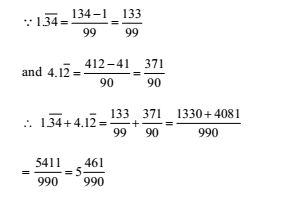Share of B + C = 1872/( 9- 3)  × (5 + 8) = Rs. 4056

Equivalent% interest for compound rate of interest of 8% for 2 years 8 +8 +(8x 8)/ 100= 16.64%

So, interest = 16.64% of 3980 = 662

1. Answer: (c)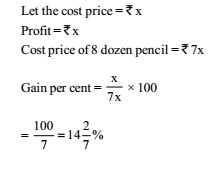2. Answer: (a)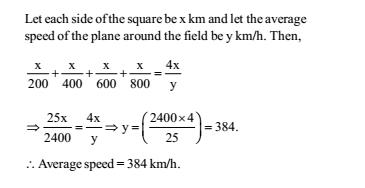A’s one day’s work =1/ 6

B’s one day’s work =1/8

C’s one day’s work =1/ 12

A’s share: B’s share: C’s share 1/ 6:1/ 8:1/ 12

Multiplying each ratio by the L.C.M. of their denominators, the ratios become 4: 3: 2

B’s share 1350x 3 /9   = 450

Ratio of equivalent capitals of A, B and C for 1 month

= 35000 × 12: 20000 × 5: 15000 × 7 = 35 × 12: 20 × 5: 15 × 7 = 84: 20: 21

Sum of the ratios = 84 + 20 + 21 = 125

B’s share = Rs. 20/125 x84125 = Rs.13460

1. Answer: (a)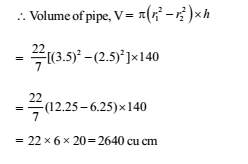xyxy = xy × 100 + xy = xy (100 + 1) = 101 × xy

Hence, the number is exactly divisible by 101.

RRB ALP 2018 – “All in One” Study Materials and Practice Sets